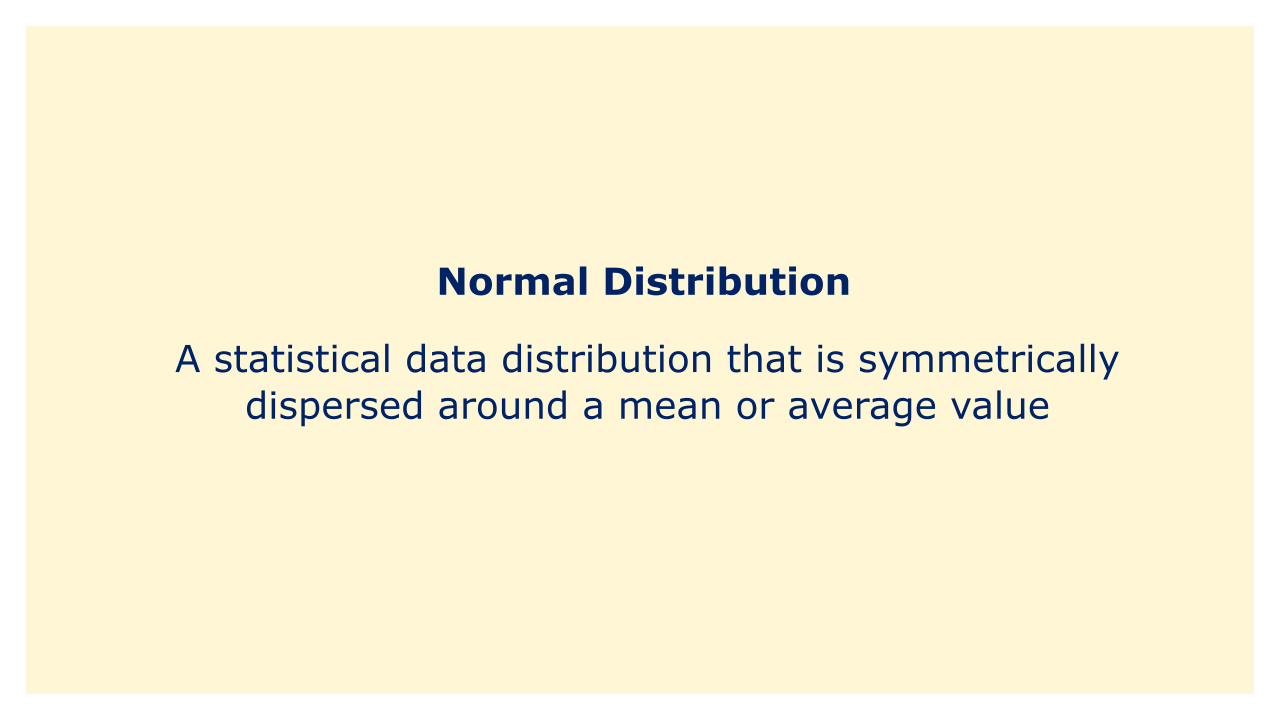# Normal DistributionImage: Moneybestpal.com

### The term "normal distribution" is used in finance to describe a statistical data distribution that is symmetrically dispersed around a mean or average value. Because of its distinctive bell-shaped curve, this form of distribution is often known as a Gaussian distribution or a bell curve.

The probability of specific outcomes, such as stock returns or interest rates, is frequently modeled in finance using the normal distribution. Two factors—the mean and the standard deviation—define the distribution. The standard deviation (or spread) of the data around the mean is represented by the standard deviation, whereas the mean shows the average value of the data set.

Being continuous and capable of taking on any value, from negative infinity to positive infinity, is one of the main characteristics of the normal distribution. Because it may shed light on the likelihood of various outcomes and the potential risk involved with particular investments, it serves as a useful tool for modeling financial data.

Numerous financial theories and models, including the capital asset pricing model (CAPM) and the efficient market hypothesis (EMH), rely on the assumption that financial returns are distributed normally. Other statistical distributions might be better suitable for some types of data, and in fact, financial data might not always follow a normal distribution.
Tags